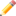## FANDOM

410 PagesThis article is a stub. You can help Programmer's Wiki by expanding it.Remember to remove this when the stub is fixed.

Foreach (or For each) loops are used for iterating over the items in a collection.

## IntroductionEdit

For loops provide an easy way to traverse arrays. as part of the loop a variable is created that is incremented after each loop is completed. This variable can be used as the index to an array as well. However if the loop is just making a modification to each item then this method is often a clumsy way of expressing the programmers intentions. The For Each loop simplifies this by exposing each item without needing to know it's position in the list.

The collection objects must support the appropriate interface (as defined by the language).

## C++Edit

```std::list<int> intList;
intList.push_back(1);
intList.push_back(7);
intList.push_back(3);

// Printing
for(std::list<int>::const_interator i = intList.begin(); i != intList.end(); ++i)
std::cout << *i << std::endl;

// Editing
for(std::list<int> i = intList.begin(); i != intList.end(); ++i)
(*i) *= 2;

// foreach_const and foreach macros
#define foreach(T, c, i) for(T::iterator i = c.begin(); i!=c.end(); ++i)
#define foreach_const(T, c, i) for(T::const_iterator i = c.begin(); i!=c.end(); ++i)

// Usage of macros
foreach_const(std::list<int>, intList, i)
std::cout << *i << std::endl;
foreach(std::list<int>, intList, i)
(*i) *= 2;
```

Limitation of macros: The type of the container cannot contain comma (`,`). Workaround like this:

```#typedef std::map<int,string> IntStringMap;
// ....
foreach_const(IntStringMap, intToString, i)
std::cout << i->first << "=" << i->second << std::endl;
```

See also `std::for_each` for a limited functionality.

## GroovyEdit

In groovy the each method can be used as a for each loop.

```[1,2,3,4].each{
println it
}
```

Also the for loop can be used on collections

```def list = [1,2,3,4]

for(item in list){
println item
}
```

## PerlEdit

```@arr = (1, 2, 3, 4, 5);
foreach my \$item (@arr) {
print \$item;
}
```

## PHPEdit

Simple foreach loop:

```// create an array containing some numbers
\$arr = array(1, 2, 3, 4, 5);
//print every number
foreach (\$arr as \$number) {
echo "\$number<br/>";
}
```

Foreach for associative array:

```// create an associative array
\$arr['name'] = "Katy";
\$arr['surname'] = "Jones":
\$arr['age'] = 29;
// print \$arr's key (name, etc) and values ("Katy", etc)
foreach (\$arr as \$key => \$value) {
echo "<strong>\$key</strong>: \$value<br/>";
}
```

## Visual BasicEdit

Any object that implements the `IEnumerable`, `ICollection`, or their generic counterparts, including arrays, can be traversed by a For Each loop.

```Dim x As New List(Of String)
For Each item As String In x
Console.Write(item)
Next
```

The type declaration can be omitted if `Option Infer` is set. In `IEnumerable` implementations, the traversal of the collection can be customized by customizing the corresponding `IEnumerator`.

## JavascriptEdit

```var item;
var x = new Array();

x = "apples";
x = "oranges";
x = "books";

for (item in x)
{
document.write(x[item] + "<br />");
}
```
1. http://www.sgi.com/tech/stl/for_each.html
Control structures
If statement - Switch statement - While loop - For loop - Foreach loop - Do while loop